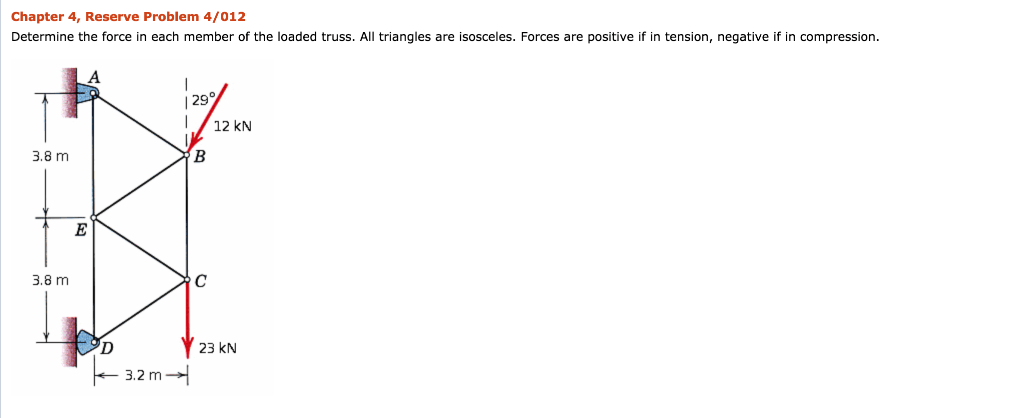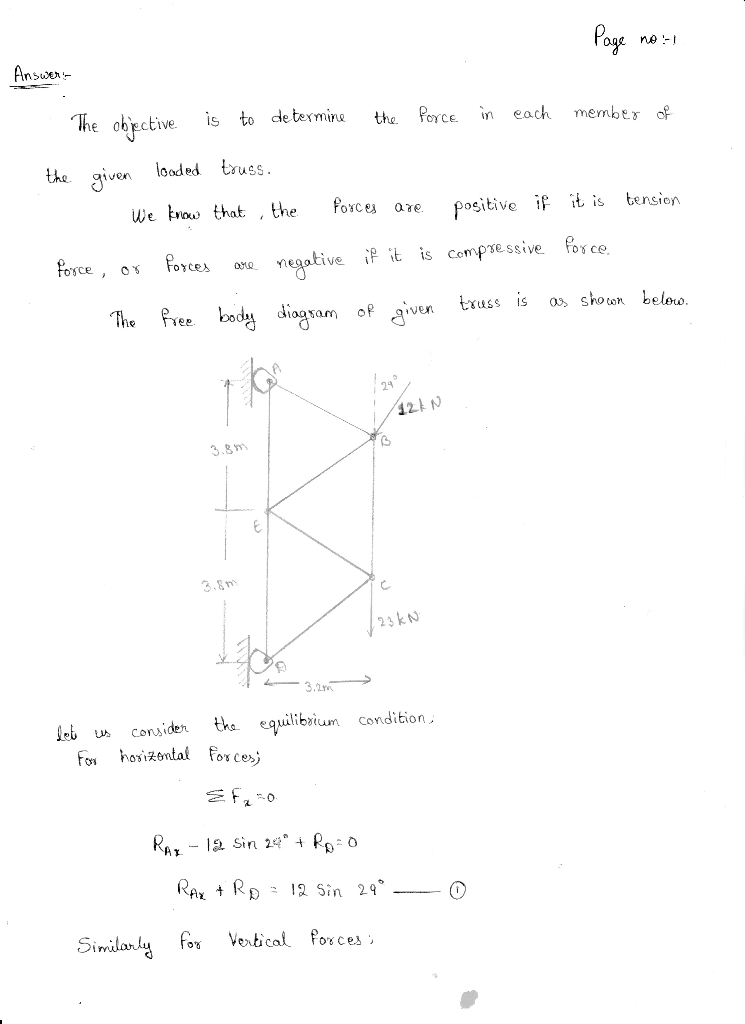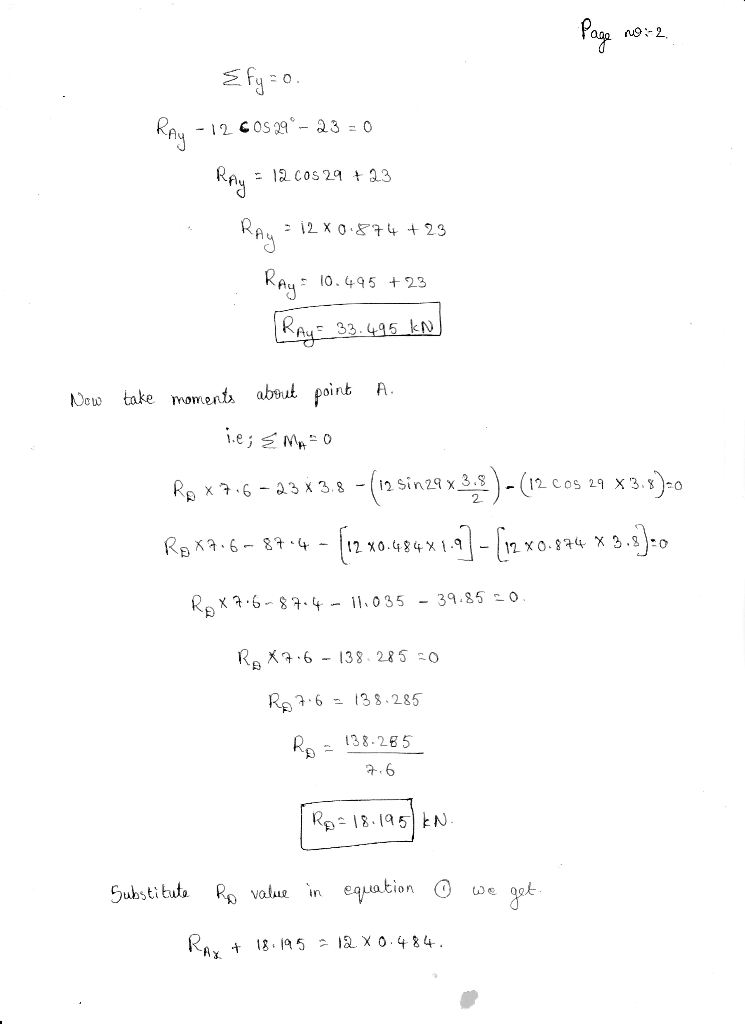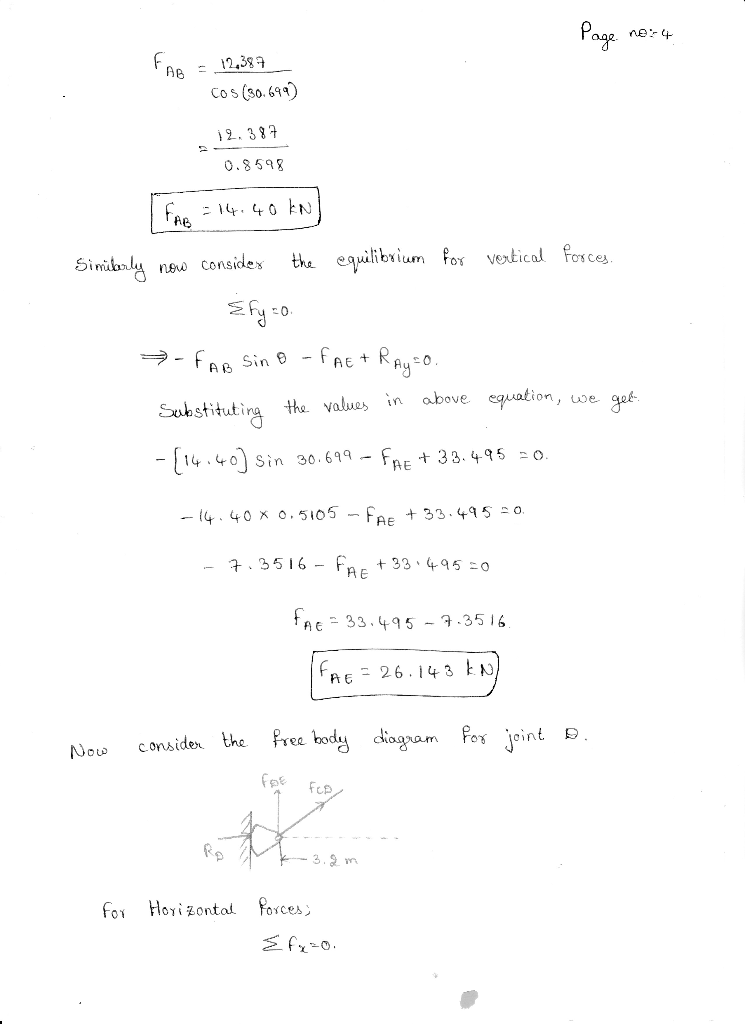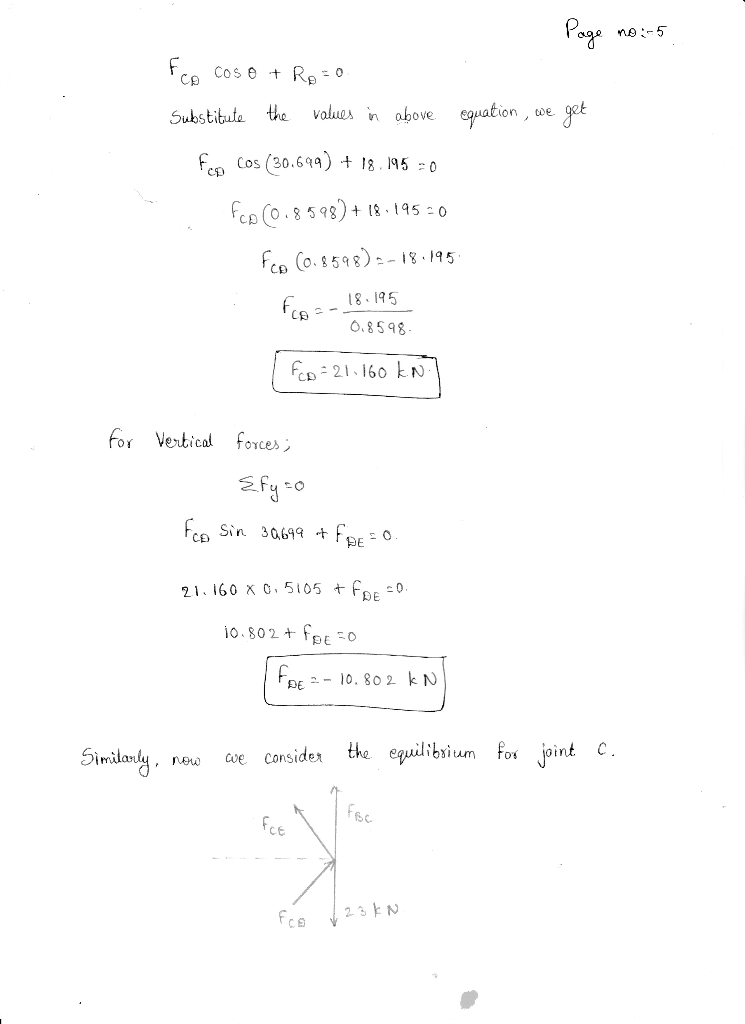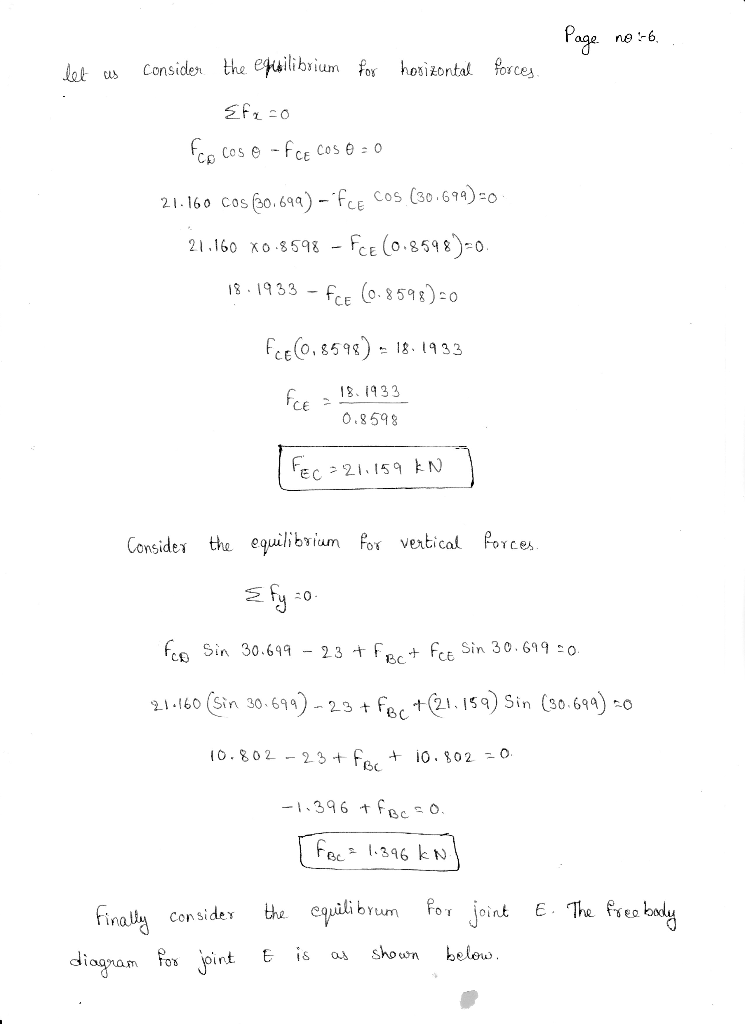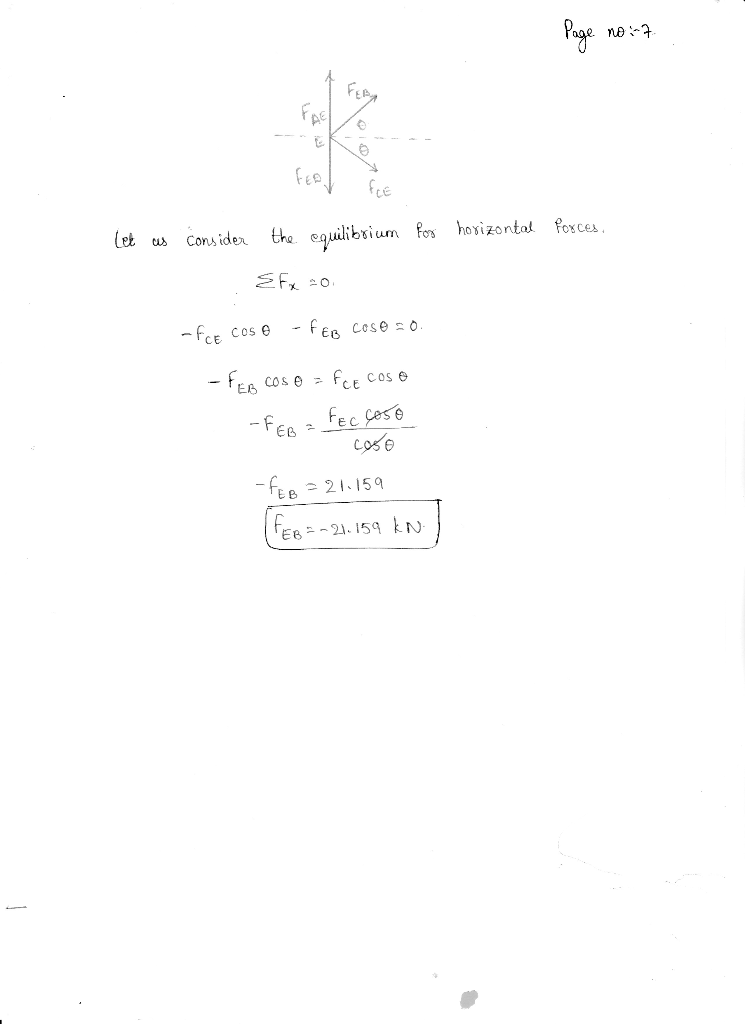# Determine the force in each member of the loaded truss. All triangles are isosceles. Forces are positive if in tension, negative if in compression.

Question-AnswerCategory: Engineering MechanicsDetermine the force in each member of the loaded truss. All triangles are isosceles. Forces are positive if in tension, negative if in compression.

Determine the force in each member of the loaded truss. All triangles are isosceles. Forces are positive if in tension, negative if in compression.